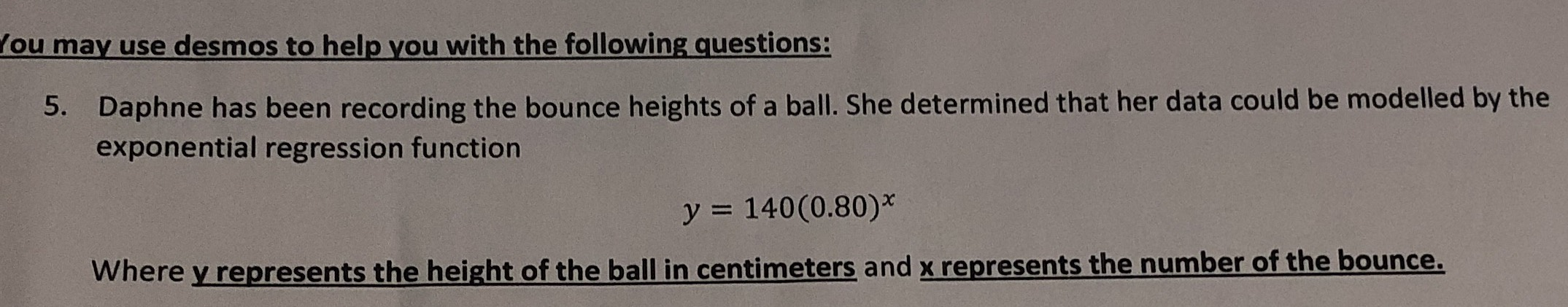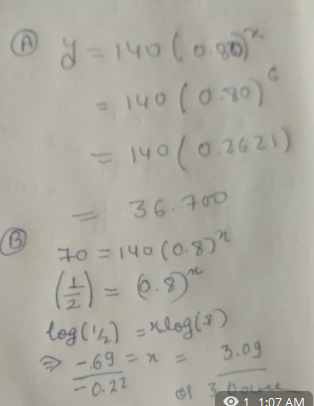### ¿Todavía tienes preguntas de matemáticas?

Pregunte a nuestros tutores expertos
Algebra
Pregunta5. Daphne has been recording the bounce heights of a ball. She determined that her data could be modelled by the exponential regression function

$$y = 140 ( 0.80 ) ^ { x }$$

Where $$y$$ represents the height of the ball in centimeters and $$\underline { x }$$ represents the number of the bounce.